# OHM’s LAW

## Electricity of Class 9

The law stating that the direct current flowing in a conductor is directly proportional to the potential difference between its ends. It is usually formulated as V = IR, where V is the potential difference, or voltage, I is the current, and R is the resistance of the conductor or  the flow of electric current through a conductor depends on the potential difference across its ends. At a particular temperature, the strength of current flowing through it is directly proportional to the potential difference across its ends. This is known as Ohm's Law.

I  ∝ V

or V ∝ I V = Potential difference

V = RI ,           Where   R = Resistance

or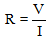where  I = Current

Here, R is the constant of proportionality, which depends on size, nature of material and temperature. R is called the electrical resistance or resistance of the conductor.

### EXPERIMENTAL VERIFICATION OF OHM'S LAW

• Set up a circuit as shown in figure consisting of a nichrome wire XY of length, say 0.5 m, an ammeter, a voltmeter and four cells of 1.5 V each. (Nichrome is an alloy of nickel, chromium, manganese, and iron metal.)
• First use only one cell as the source in the circuit. Note the reading in the ammeter I, for the current and reading of the voltmeter V for the potential difference across the nichrome wire XY in the circuit. Tabulate them.
• Next connect two cells in the circuit and note the respective readings of the ammeter and voltmeter for the values of current through the nichrome wire and potential difference across the nichrome wire.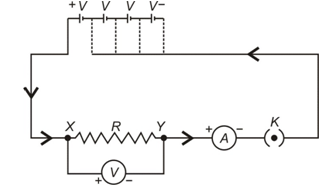• Repeat the above steps using three cells and then four cells in the circuit separately.

• Calculate the ratio of V to I for each pair of potential difference (V) and current (I).
• Plot a graph between V and I, and observe the nature of the graph.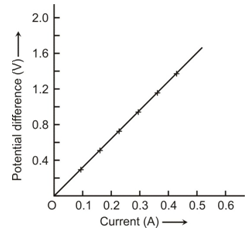Thus,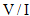is a constant ratio which is called resistance (R). It is known as Ohm’s Law.

### RESISTANCE OF A CONDUCTOR:

There are three external factors that influence the resistance in a conductor.

• Thickness (cross sectional area of the wire),
•  Length
•  Temperature

All have some effect on the amount of resistance created in a conductor.  The fourth factor is the conductivity of the material we are using.  Some metals are just more electrically conductive than others.  This however, is considered an internal factor rather than an external one.

The electric current is a flow of electrons through a conductor. When the electrons move from one part of the conductor to the other part, they collide with other electrons and with the atoms and ions present in the body of the conductor. Due to these collisions, there is some obstruction or opposition to the flow of electrons through the conductor.

The property of a conductor due to which it opposes the flow of current through it, is called resistance. The resistance of a conductor is numerically equal to the ratio of potential difference across its ends to the current flowing through it.

Resistance =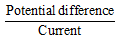Or R =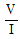### Cross Sectional Area

The cross-sectional area of a conductor (thickness) is similar to the cross section of a hallway.  If the hall is very wide, it will allow a high current through it, while a narrow hall would be difficult to get through due to it's restriction to a high rate of flow.  The animation at the left demonstrates the comparison between a wire with a small cross sectional area (A) and a larger one (A).  Notice that the electrons seem to be moving at the same speed in each one but there are many more electrons in the larger wire. This results in a larger current which leads us to say that the resistance is less in a wire with a larger cross sectional area.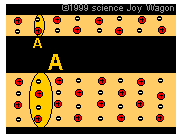• Length of the Conductor: The length of a conductor is similar to the length of a hallway.  A shorter hallway would allow people to move through at a higher rate than a longer one.
• Temperature: The temperature of a conductor has a less obvious effect on the resistance of the conductor.  It would be as hard to apply the hallway analogy as it is hard to say whether a hot hallway would make us move faster or slower than a cold hallway.  To truly understand the effect you must picture what happens in a conductor as it is heated. Heat on the atomic or molecular scale is a direct representation of the vibration of the atoms or molecules.  Higher temperature means more vibrations. Since the wire is cold the protons are not vibrating much so the electrons can run between them fairly rapidly.  As the conductor  heats up, the protons start vibrating and moving slightly out of position.  As their motion becomes more erratic they are more likely to get in the way and disrupt the flow of the electrons. As a result, the higher the temperature, the higher the resistance. A prime example of this is when you turn on a light bulb. The first instant, the wire (filament) is cold and has a low resistance but as the wire heats up and gives off light it increases in resistance.   As a result we can say that Ohm's law holds true unless temperature changes.At extremely low temperatures, some materials have no measurable resistance. This is called superconductivity. The materials are known as superconductors. We are creating materials that become superconductors at higher temperatures and the race is on to find or create materials that superconduct at room temperature.  We are painfully far away from the finish line.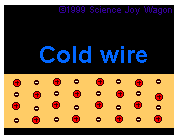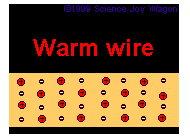### UNIT OF RESISTANCE

The S.I. unit of resistance is Ohm (Ω)

1 Ohm (Ω) =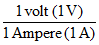The resistance of a conductor is said to be one ohm if a current of one ampere flows through it when a potential difference of one volt is applied across its ends.

### CONDUCTORS, RESISTORS AND INSULATORS:

We have already study this in flow of charge form. But in different sense in terms of electricity we know this

On the basis of their electrical resistance, all the substances can be divided into three groups:

conductors, resistors and insulators.

• Conductors: Those substances which have very low electrical resistance are called conductors. A conductor allows the electricity to flow through it easily. Silver metal is the best conductor of electricity Copper and Aluminium metals are also good conductors. Electric wires are made of Copper or Aluminium because they have very low electrical resistance.

`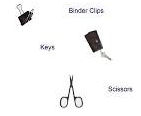• Resistors: Those substances which have comparatively high electrical resistance, are called resistors. The alloys like nichrome, manganin and constantan (or ureka), all have quite high resistances, so they are used to make those electrical devices where high resistance is required. A resistor reduces the current in the circuit.
•  Insulators:Those substances which have infinitely high electrical resistance are called insulators. An insulator does not allow electricity to flow through it. Rubber is an excellent insulator. Electricians wear rubber handgloves while working with electricity because rubber is an insulator and protects them from electric shocks. Wood is also a good insulator.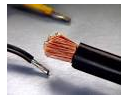Activity to show that the amount of current through an electric component depends upon its resistance:

• Take a nichrome wire, a torch bulb, a 10 W bulb and an ammeter (0-5 A range), a plug key and some connecting wires.
• Set up the circuit by connecting four dry cells of 1.5 V each in series with the ammeter leaving a gap XY in the circuit, as shown in figure.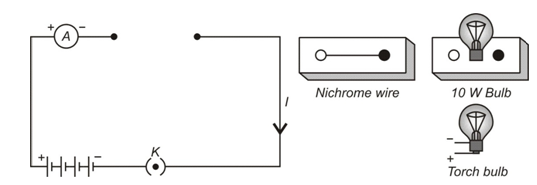• Complete the circuit by connecting the nichrome wire in the gap XY. Plug the key. Note down the ammeter reading. Take out the key from the plug. [Note: Always take out the key from the plug after measuring the current through the circuit.]
• Replace the nichrome wire with the torch bulb in the circuit and find the current through it by measuring the reading of the ammeter.
• Now repeat the above step with 10 W bulb in the gap XY.
• You will notice that the ammeter readings differ for different components connected in the gap XY.
• You may repeat this Activity by keeping any material component in the gap. Observe the ammeter readings in each case. Analyse the observations.
• Thus, we come to a conclusion that current through an electric component depends upon its resistance.

### FACTORS AFFECTING RESISTANCE OF A CONDUCTOR

Resistance depends upon the following factors:-

• Length of the conductor.
• Area of cross-section of the conductor (or thickness of the conductor).
• Nature of the material of the conductor.
• Temperature of the conductor.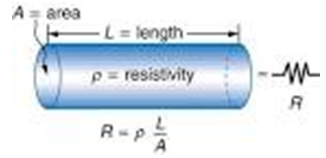This same factor we have to see mathematicaily:  It has been found by experiments that:

(i) The resistance of a given conductor is directly proportional to its length i.e.

R ∝ l ….(i)

(ii) The resistance of a given conductor is inversely proportional to its area of cross-section i.e.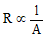….(ii)

From (i) and (ii),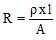…(iii)

Where ρ (rho) is a constant known as resistivity of the material of the conductor. Resistivity is also known as specific resistance.

Experiment to show that resistance of a conductor depends on its length, cross section area and nature of its material.

• Complete an electric circuit consisting of a cell, an ammeter, a nichrome wire of length [marked (1)] and a plug key, as shown in figure.
• Now, plug the key. Note the current in the ammeter.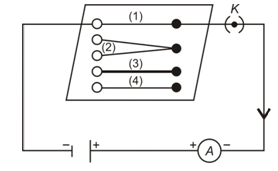• Replace the nichrome wire by another nichrome wire of same thickness but twice the length, that is 2 [marked (2) in the figure]. Note the ammeter reading.
• Now replace the wire by a thicker nichrome wire, of the same length  [marked(3)]. A thicker wire has a larger cross-sectional area. Again note down the current through the circuit.
• Instead of taking a nichrome wire, connect a copper wire [marked (4) in figure] in the circuit. Let the wire be of the same length and same area of cross-section as that of the first nichrome wire [marked(1)]. Note the value of the current.
• Notice the difference in the current in all cases.
• We notice that the current depends on the length of the conductor.
•  We also observed that the current depends on the area of cross-section of the wire used.

### RESISTIVITY:

We will define resistivity by the help of its formula as

Resistivity,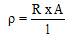….(iv)

By using this formula, we will now obtain the definition of resistivity. Let us take a conductor having a unit area of cross-section of 1 m2 and a unit length of 1 m. So, putting A= 1 and l = 1 in equation (iv),

we get:

Resistivity, ρ = R

The resistivity of a substance is numerically equal to the resistance of a rod of that substance which is 1 metre long and 1 metre square in cross-section.

Unit of resistivity,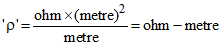The S.I. unit of resistivity is ohm-metre which is written in symbols as Ω - m.

 Material Resistivity /Ωm Copper 1.7 × 10-8 Aluminum 2.7 × 10-8 Graphite 8.0 × 10-6 Silicon 2.3 × 103 Quartz 8.0 × 1016

Resistivity of a substance does not depend on its length or thickness. It depends only on the nature of the substance. The resistivity of a substance is its characteristic property. So, we can use the resistivity values to compare the resistances of two or more substances.

• Resitivity is a measure of the resistance to electrical conduction for a given size of material. Its opposite is electrial conductivity (=1/resistivity).
• Metals are good electrical conductors (high conductivity and low resistivity), while non-metals are mostly poor conductors (low conductivity and high resistivity).
• The more familiar term electrical resistance measures how difficult it is for a piece of material to conduct electricity - this depends on the size of the piece: the resistance is higher for a longer or narrower section of material.
• To remove the effect of size from resistance, resistivity is used - this is a material property which does not depend on size.
• Restivity is affected by temperature - for most materials the resistivity increases with temperature. An exception is semiconductors (e.g. silicon) in which the resistivity decreases with temperature.
• The ease with which a material conducts heat is measured by thermal conductivity. As a first estimate, good electrical conductors are also good thermal conductors.

### SPECIFIC USE OF SOME CONDUCTING MATERIALS:

Tungsten: It has high melting point of 3380ºC and emits light at 2127ºC. It is thus used as a filament in bulbs.

Nichrome: It has high resistivity and melting point. It is used as an element in heating devices.

Constantan and Manganin: They have modulated resistivity. Thus they are used for making resistances and rheostats.

Tin-lead Alloy: It has low resistivity and melting point. Thus it is used as fuse wire.

Ex. When a 12 V battery is connected across an unknown resistor, there is a current of 2.5 mA in the circuit. Find the value of the resistance of the resistor.

Solution: Given that voltage of battery V = 12 V

Circuit current I = 2.5 mA = 2.5 ×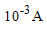∴ Value of resistance R =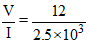= 4800 Ω